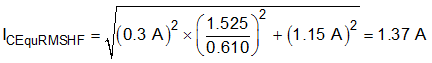SLUSD37E October   2017  – November 2019

PRODUCTION DATA.

1. Features
2. Applications
3. Description
1.      Device Images
4. Revision History
5. Device Comparison Tables
6. Pin Configuration and Functions
7. Specifications
8. Detailed Description
1. 8.1 Overview
2. 8.2 Functional Block Diagram
3. 8.3 Feature Description
1. 8.3.1 CrM/DCM Control Principle
2. 8.3.2 Line Voltage Feed-Forward
3. 8.3.3 Valley Switching and CrM/DCM Hysteresis
4. 8.3.4 Transconductance Amplifier with Transient Speed-up Function
5. 8.3.5 Faults and Protections
6. 8.3.6 High-Current Driver
4. 8.4 Controller Functional Modes
9. Application and Implementation
1. 9.1 Application Information
2. 9.2 Typical Application
1. 9.2.1 Design Requirements
2. 9.2.2 Detailed Design Procedure
1. 9.2.2.1 Custom Design With WEBENCH® Tools
2. 9.2.2.2 Power Stage Design
3. 9.2.2.3 ZCD/CS Pin
4. 9.2.2.4 VOSNS Pin
5. 9.2.2.5 Voltage Loop Compensation
3. 9.2.3 Application Curves
10. 10Power Supply Recommendations
11. 11Layout
1. 11.1 Layout Guidelines
2. 11.2 Layout Example
12. 12Device and Documentation Support
13. 13Mechanical, Packaging, and Orderable Information

• DBV|6

#### 9.2.2.2.4 Output Capacitor Selection

Power drawn by the PFC stage from the Line supply may be represented by the following expression.

Equation 39.Assuming a typical application, with constant load power, for some parts of the Line cycle excess power is drawn from the supply and stored in the output capacitor. In other parts of the Line cycle load power exceeds input power and this deficit must be supplied from the output capacitor. This process of energy transfer to an from the output capacitor necessarily results in twice Line frequency output voltage ripple. The amplitude of this twice Line frequency ripple depends only upon the ratio POut/COut and the Line frequency.

Equation 40.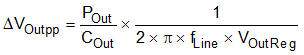Choose an output capacitor value by prioritizing one of a number of application requirements:

• Twice Line frequency output ripple voltage at maximum load.
• Output voltage hold-up time after the Line supply has been disconnected.
• Output voltage deviation as a result of a transient load step.

For this design example assume that the twice Line frequency output ripple voltage amplitude is less than 3% of its regulation level. The POutMax/COut ratio required to achieve this can be calculated using Equation 41

Equation 41.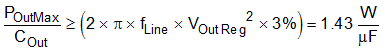Use Equation 42 to calculate the required capacitance value for this 165-W example design.

Equation 42.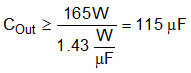For best Line current total harmonic distortion (THD), the maximum output voltage ripple amplitude must satisfy the condition presented in Equation 43. Satisfying this condition ensures that the error amplifier non-linear gain does not activate due to extremes of the output voltage ripple.

Equation 43.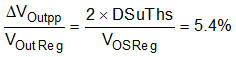Use Equation 44 to calculate the maximum RMS ripple current flowing in the output capacitor.

Equation 44.This current flowing into the output capacitor includes a switching frequency component (ICOutRMSHF) and a twice Line frequency ripple component (ICOutRMSLF).

Equation 45.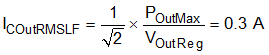Equation 46.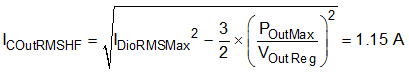Electrolytic capacitors typically have a ripple current rating at twice Line frequency (120 Hz) and a different ripple current rating at switching frequency (100 kHz). These ratings reflect the fact that the capacitor ESR is higher at twice Line frequency and hence ripple current at this frequency leads to higher power loss than the same amplitude of switching frequency ripple. Consider the equivalent high-frequency ripple current flowing in the capacitor in order to select the correct capacitor.

Equation 47.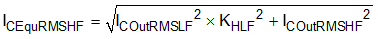The parameter KHLF is the ratio of high frequency to low frequency RMS ripple current rating for the particular capacitor series to be used.

Equation 48.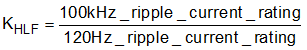In this example design, for reasons of size and rating, two 68-µF, 450 V capacitors are selected from Rubycon BXW series (450BXW68MEFC12.5X45), connected in parallel. In this way, both the capacitance value requirement and ripple current rating are met with some additional margin.

Equation 49.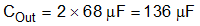Equation 50.